# User:IssaRice/Linear algebra/Classification of operators

Let$V$ be a finite-dimensional inner product space, and let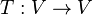$T : V \to V$ be a linear transformation. Then in the table below, the statements within the same row are equivalent.$T$ is diagonalizable There exists a basis of$V$ consisting of eigenvectors of$T$$T$ is diagonalizable (there exists a basis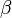$\beta$ of$V$ with respect to which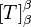$[T]_\beta^\beta$ is a diagonal matrix) This basis is not unique because we can reorder the vectors and also scale eigenvectors by a non-zero number to obtain an eigenvector. But there are at most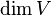$\dim V$ distinct eigenvalues so the diagonal matrix should be unique up to order? If$T$ is the identity map, then every non-zero vector$v \in V$ is an eigenvector of$T$ with eigenvalue$1$ because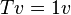$Tv = 1v$. Thus every basis$\beta = (v_1,\ldots,v_n)$ diagonalizes$T$. The matrix of$T$ with respect to$\beta$ is the identity matrix.$T$ is normal There exists an orthonormal basis of$V$ consisting of eigenvectors of$T$$T$ is diagonalizable using an orthonormal basis$T$ self-adjoint ($T$ is Hermitian) There exists an orthonormal basis of$V$ consisting of eigenvectors of$T$ with real eigenvalues$T$ is diagonalizable using an orthonormal basis and the diagonal entries are all real$T$ is an isometry There exists an orthonormal basis of$V$ consisting of eigenvectors of$T$ whose eigenvalues all have absolute value 1$T$ is diagonalizable using an orthonormal basis and the diagonal entries all have absolute values 1 This only works when the field of scalars is the complex numbers$T$ is positive There exists an orthonormal basis of$V$ consisting of eigenvectors of$T$ with positive real eigenvalues$T$ is diagonalizable using an orthonormal basis and the diagonal entries are all positive real numbers